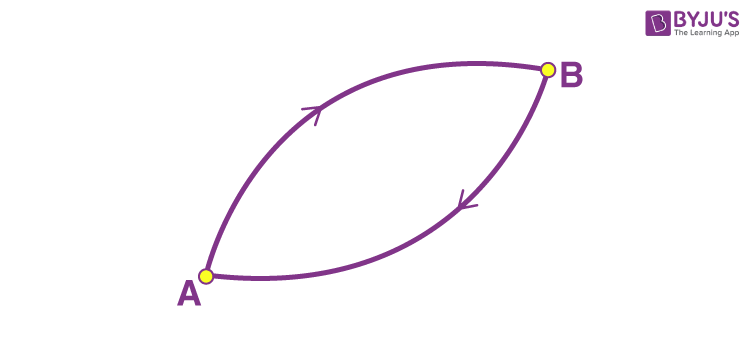# Prove Electric Field Is Conservative In Nature

The electric field is defined as the electric force per unit charge. If we observe the electric field pattern, it is radially directed outward from a positive charge and is directed inwards a negative point charge. The electric field is a vector quantity, and the SI unit of the electric field is volts per meter. The electric field is a conservative field. Let us know what is meant by conservative field and how to prove the electric field is conservative in nature.

A force is said to be conservative if the work done by the force in moving a particle from one point to another point depends only on the initial and final points and not on the path followed.

The field where the conservative force is observed is known as a conservative field.

Example: Consider an electric field created due to a charge Q. The work done to carry a test charge (q) from point A to another point B in the field due to Q does not depend upon the path followed. Electric field depends upon the initial and final positions A and B. Electric fields are independent of the path followed. So we say that the electric field is conservative in nature.

## To Prove Electric Field Is Conservative In Nature

Consider a charge Q placed in an electric field placed in points A and B. A to B is a closed path. Work is done by the electric field to move a test charge from point A to B. To find the work done, sum up the work done for all the small segments into which the path A and B is divided. Such an integral evaluated along a line is called a line integral.The line integral of the electric field along A to B

$\int_{A}^{B}\vec{E}.\vec{dl}$

The line integral of the electric field along B to A

$\int_{B}^{A}\vec{E}.\vec{dl}$

Hence, the line integral along the closed path as shown in the figure above is given by

$\int_{A}^{B}\vec{E}.\vec{dl} + \int_{B}^{A}\vec{E}.\vec{dl}$

But,

$\int_{B}^{A}\vec{E}.\vec{dl} = – \int_{A}^{B}\vec{E}.\vec{dl} \int_{A}^{B}\vec{E}.\vec{dl} + \int_{B}^{A}\vec{E}.\vec{dl} = \int_{A}^{B}\vec{E}.\vec{dl} + – \int_{A}^{B}\vec{E}.\vec{dl}$

This shows that the line integral of an electric field along a closed path is zero.

Hence, the work done by the electric field is independent of the path, which means it depends only on points A and B.

Hence we can prove that the electric field is conservative in nature.

Stay tuned with BYJU’S for more such interesting derivations in physics, chemistry and maths in an engaging way with video explanations.

## Frequently Asked Questions on Work

### 1. What is work?

Work is defined as the product of the force in the direction of the displacement and the magnitude of the displacement.

### 2. What is the SI unit of work?

SI unit of work is Joule.

Yes.

Vector quantity.

### 5. What is a conservative field?

The field where the conservative force is felt is called conservative field.